Next: Fourier transform of a Up: Properties of Fourier transforms Previous: Fourier transform of DC   Contents   Index

## Shifts and phase changes

Section 7.2 showed how time-shifting a signal changes the phases of its sinusoidal components, and Section 8.4.3 showed how multiplying a signal by a complex sinusoid shifts its component frequencies. These two effects have corresponding identities involving the Fourier transform.

First we consider a time shift. If, as usual, is a complex-valued signal that repeats everysamples, let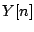bedelayedsamples:which also repeats everysamples sincedoes. We can reduce the Fourier transform ofthis way: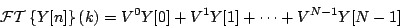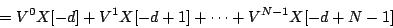(The third line is just the second one with the terms summed in a different order). We therefore get the Time Shift Formula for Fourier Transforms:The Fourier transform of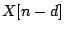is a phase term times the Fourier transform of. The phase is changed by, a linear function of the frequency.

Now suppose instead that we change our starting signalby multiplying it by a complex exponentialwith angular frequency: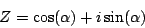The Fourier transform is:We therefore get the Phase Shift Formula for Fourier Transforms:Next: Fourier transform of a Up: Properties of Fourier transforms Previous: Fourier transform of DC   Contents   Index
Miller Puckette 2006-12-30